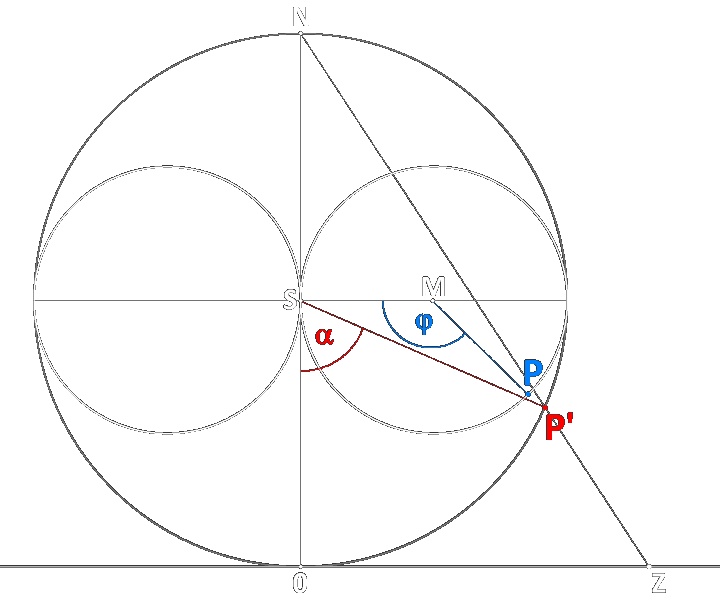conformal mapping from complex plane → Riemann sphere → horn torus, Z → P' → P or |z| → α → φ, when |z| and α are zero at south pole 0  and angle φ is measured as shown in the sketch on this page, the simpler and more consistent version (in the first considerations I had chosen α = 0 at north pole N)  stereographic projection from complex plane to Riemann sphere, |z| → α:   α = 2·arctan(|z|)  mapping from Riemann sphere to horn torus, α → φ  (C any real number*):   φ = 2·arccot(-ln(|tan(α/2)|) - C)  mapping from complex plane to horn torus, |z| → φ:   φ = 2·arccot(-ln(|z|) - C)  inverse mappings P → P' → Z, equivalent φ → α → |z|:   α = 2·arctan(e^(-cot(φ/2) + C))  |z| = tan(α/2)  |z| = e^(-cot(φ/2) + C)  ⇐   preceding page derivation of formulas (analytically by the method of infinitesimal circles)  ←  excel chart for numerical computation of angles and |z|  (15 decimal places) a graphical method (stereographic projection) Z → P  probably does not exist Vyacheslav Puha spotlights that e lies on 'top',  1/e on 'bottom' of horn torus but note: that's only valid for C = 0, one of infinite many conformal mappings it's not sure whether conformality has any relevance in the horn torus model example for a mapping that preserves right angles between coordinates only *)  C seems to be a kind of 'zoom factor' for the mappings, diminishing  projected figures and, for C > 0, moving α and φ towards π resp. 2π,  for C < 0 towards 0 both.  Likewise, but then for C > 1 resp. 0 < C < 1  (C = 1 for the stereographic projection), there exists the well known  generalised conformal mapping between complex plane and sphereα = 2·arctan(C·|z|)  resp.  |z| = tan(α/2) / CRiemann sphere:  0N = 1 ,  ∠0NP' = α/2 ,  ∠0ZN = π/2 - α/2 ,  |z| = 0Z   gif    conformal mapping sphere ↔ horn torus

home  despite these webpages contain mathematical formulas, the intention of the site neither is mathematical nor physical - it's a game!  Background is critical philosophy combined with spirit of (mental) adventure and enjoyment of (digital)   arts